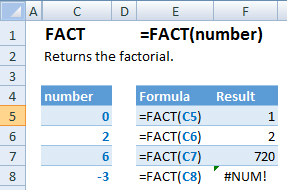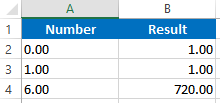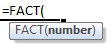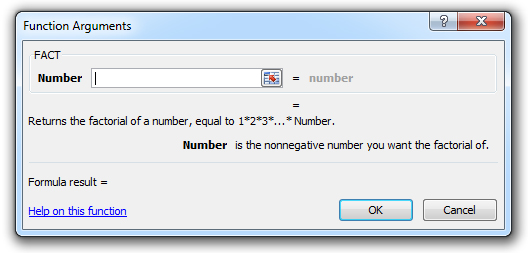### FACT Function – Calc Factorial in Excel, VBA & Google Sheets

This Excel Tutorial demonstrates how to use the Excel FACT Function in Excel to return the factorial, with formula examples.

## FACT Function Description:

The FACT Function Returns the factorial.

## Formula Examples:Example Formula Result
1 =FACT(C5) 1
2 =FACT(C6) 2
3 =FACT(C7) 720
4 =FACT(C8) #NUM!
AutoMacro - VBA Code Generator

## Syntax and Arguments:

The Syntax for the FACT Formula is:

`=FACT(number)`

### Function Arguments ( Inputs ):

number – A number.

## Additional Notes

Use the FACT Functino to calculate the factorial for a given number. Simply enter any number directly into the formula, or reference a cell containing a number.

## FACT Examples in VBA

You can also use the FACT function in VBA. Type:
`application.worksheetfunction.fact(number)`

Executing the following VBA statements

```Range("B2") = Application.WorksheetFunction.Fact(Range("A2"))
Range("B3") = Application.WorksheetFunction.Fact(Range("A3"))
Range("B4") = Application.WorksheetFunction.Fact(Range("A4"))```

will produce the following resultsFor the function arguments (number, etc.), you can either enter them directly into the function, or define variables to use instead.

Return to the List of all Functions in Excel

## How to use the FACT Function in Excel:

To use the AND Excel Worksheet Function, type the following into a cell:
`=AND(`
After entering it in the cell, notice how the AND formula inputs appear below the cell:You will need to enter these inputs into the function. The function inputs are covered in more detail in the next section. However, if you ever need more help with the function, after typing “=FACT(” into a cell, without leaving the cell, use the shortcut CTRL + A (A for Arguments) to open the “Insert Function Dialog Box” for detailed instructions:For more information about the FACT Formula visit the
Microsoft Website.

## Excel Practice Worksheet

Practice Excel functions and formulas with our 100% free practice worksheets!

• Automatically Graded Exercises
• Learn Excel, Inside Excel!